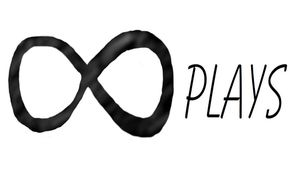By Bob

Just for fun I decided to try and make a module for drone propellers, as it progressed I was also able to use the customizer to make impeller designs and all types of different  experimental versions.

Disclaimer: I have not tested any of the designs and I have no idea if they will work, this was an exercise in experimenting with OpenScad code to see what I could come up with and it is strictly for educational, fun and personal use. Since it only took me an hour to come up with this it will have errors<feel free to make improvements.

If you find this module useful a link back to this site and attribution would be great.

Here is the code just copy and paste it into the Opencad editor:

``````Show_impeller = true;
Diameter=80; //[1:.01:200]
Fins = 6; //[2:1:20]
Twist= 120; //[-360:.01:360]
Scale_x=1; //[.2:.01:20]
Scale_y=1.88; //[.2:.01:20]
Scale_z=.17; //[.1:.01:20]
Rotate_x=0; //[0:.01:360]
Rotate_y=66.15; //[0:.01:360]
Rotate_z=123.39; //[0:.01:360]
Fin_hoop_width=200; //[.1:.01:200]
Center_hub_size=22.26;//[0:.01:50]
Center_hole_size=5;//[0:.01:50]
Scale_center_x=1; //[-.25:.01:20]
Scale_center_y=1; //[-.25:.01:20]
Scale_center_z=1.75; //[-.25:.01:20]

module impeller(){
if(Show_impeller)
for (i=[1:Fins]){
rotate([0,0,(360/Fins)*i])
difference(){
scale([Scale_x,Scale_y,Scale_z])
intersection(){
translate([0,0,-Diameter/2])
linear_extrude(Diameter,twist=Twist,slices=360,convexity = 10)
rotate([Rotate_x,Rotate_y,Rotate_z])
sphere(d=Diameter,\$fn=100);
}
scale([Scale_x,Scale_y,Scale_z])
sphere(d=(Diameter-Fin_hoop_width),\$fn=100);
cylinder(d=Center_hub_size-12,h=Diameter+2,center=true,\$fn=100);
}
difference(){
scale([Scale_center_x,Scale_center_y,Scale_center_z]){
sphere(d=(Center_hub_size),\$fn=100);
}
cylinder(d=Center_hole_size,h=200,center=true,\$fn=100);
}}
}

impeller();``````